A Different Perspective

Part VI section b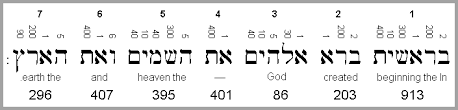To get an idea of the complexity of the Torah, we need look no further than its first verse, which has exactly 127 different combinations of its 7 words. This is considering only variations in which of the 7 words are being added together, not their order. If we were to consider rearranging their order (permuting them), or their 28 individual letters, their possible combinations would be ridiculously high. Nonetheless, 127 is the 7th Mersenne Prime.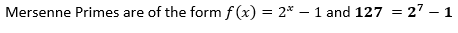The sum of those 127 assorted combinations is 172864. Where did we see that number before?

It was in the total gematria of the collective 304,805 letters in the Torah: 21009826. The sum of its digits was (2+1+0+0+9+8+2+6) = 28, and the product of those same digits gave us (2*1*0*0*9*8*2*6) = 1728.  Then we applied a deeply revealing form of gematria cipher and got an equation which circled us back to the 28 letters of the Torah’s first verse:

4√(P(21009826)/ ∑(21009826))) 4√(1728/28) = 2.802828

Now with the sum of those 127 assorted combinations equal to 172864, the relevance between the two equations and the entirety of the Torah to its first verse is strengthened.

Within those 127 or 27 combinations each word (value) is represented 64 or 26 times, which also means 172864/2701 (the value of the first verse) equals 64.  Moreover, while 2701 = (73 x 37), the total 127 combined combinations, or 172864, work out to both 4672 x 37 and 2368 x 73.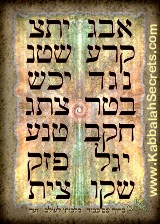It may be telling that 2368 was the year Moses was born, yet there is another property to these numbers, a geometric one. An odd synergy or linkage is found between the these total combinations and the 42-Letter Name matrix. When we add 4672 and 2368 together, we get 7040, and when we divide the sum of all 64 value 407’s by 37 we also get exactly 704. As it turns out 704 is the value of the 4th level of the 7 levels of the 42-Letter Name matrix.  Kabbalistically, this is the level connected to Moses.

As for the number 1728 above, there are 7 words and 28 letters in the Torah’s first verse and the longest word in the Torah (ובמשארותיך) is found at Exodus 7:28. This unique word (ובמשארותיך) is 10 letter long, and in reverse order, the word, keter (כתר) and the acronym for the 42-Letter Name (שמב) are easily found . We are keying in on this word specifically, not for what it means but because it is unique in being 10 letters long and found in the 10 plagues. Utilizing the 4 remaining letters, they spell both Ohr(אור), meaning light, and the number 216 (ריו), as in the 216 letters to be found 7 verses later in the 72 Triplets. Furthermore, the first 4 letters of this unique word have an ordinal value of 42 and the remaining 6 letters of 70. We can speculate all day as to why these hidden gems are found in the “kneading bowls” of the Egyptians during the 2nd plague, but all we do know is that every gem networks to another one and another and all these interconnections appear to form a neural network of sorts and also a roadmap of intelligent design, like keter(620) in this 10-letter word connecting to the 10 Commandments comprised of 620 letters at the high point (keter) of the Torah in the 70th chapter of the Torah.

Moreover, there are 728 consecutive-word pairs in the Torah that begin with the letters Mem(מ) and Bet(ב), as in the Mem-bet (מב) or 42-Letter Name. The more we look, the more all those neural connections seem to be tied into the 42-Letter Name, which may be why the Torah blatantly told us the Israelites had to go through 42 (מב) journeys, each described with the prefixes Mem(מ) and Bet(ב) in order to get to the Promised Land. That message could not have been clearer. Are we reading the hidden messages loud and clear as well?

If an advanced intelligence gave us a roadmap of intelligent design could it be just a gigantic Rubik cube to stimulate our minds—not a bad thing in and of itself? Or since we were instructed to preserve it, study it, obey its laws, and read it weekly forever could we assume that it was something much more significant for us? What about if that advanced intelligence was Divine intelligence?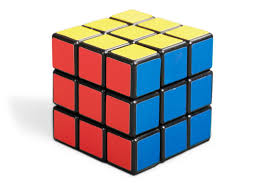If this intelligent artifact were found on the side of the road somewhere it could indeed be a left behind Rubik’s cube from an intergalactic garden party, but we know that is not the case.

If we take this intelligently designed document/matrix at face value, Moses was given 173 keys to Heaven, akin to the 173 value of the 42-Letter Name, and was able to download the entire Torah into his mind, all 58 element, or even 248,000Φ elements, and recreate it absolutely perfectly. He also downloaded the designs for the Tabernacle, the Ark of the Covenant, and much more. The Torah takes pains to tell us Moses was a man of specific human origin, not a divine being or a mysterious being who appeared out of nowhere. Is it possible we are being shown how much more our minds can expand and handle? Is it possible we are being shown how?

The Torah also tells us we were given the Torah from love, and gematria tells us that love (13) and Oneness(13) are synonymous.  Again, taking the word of the Torah at face value, might not that mean that this Advanced Intelligence, Divine or otherwise, wants all of us to be able to expand our minds and to free us from our limitations, the limitations bounded by what we collectively perceive as reality.

How interesting that throughout time certain historically evil forces have sought to destroy the Torah and the people who preserve and study it. Despite them and despite overwhelming odds it remains whole and intact for us today.

Also, taking the Torah’s words at face value, it is specifically and repeatedly—several thousand times—telling us that this advanced intelligence is specifically Divine Intelligence. Perhaps it was always known that more than any other message this one about Divine Intelligence would have to be hammered home since it would be the hardest one for us to accept.

Cubes

Whether we take the number 1728 independently or split apart the number 172864 into 1728 and 64 we see that 1728 = 123 is a perfect cube of 12 x 12 x 12, and that 64 = 4 x 4 x 4, another perfect cube of 43. Moreover, since 1728/64 = 27 we can fit exactly 27, or 33 cubes of 43 into the cube of 123 conveniently matching the 27 building blocks in the alphabet when the 5 final letters are included.

Since each word-value is included 64 or 43 times they each form a cube of 4 units by 4 units by 4 units. So we have 7 cubes, each with 64 blocks, with the volume of each block worth each word’s face value. For example, the first cube is comprised of 64 blocks of 913 each arranged in 4 layers of 4 x 4 blocks per side. In total that give us 7 x 64 or 448 blocks (cubes)

There is also the 43 cube of 64 individual cubes of 2701 each, given that 172864/2701 = 64 = 43.

Each unit length for each side of the individual cubes are given by the cube root of each word value or (9.701158, 5.87713, 4.41400, 7.3742, 7.33723, 4.108, and 6.66444) respectively. We’ll get to these values in due time. Just note that each side of the 43 cube based on the 7th word “The Earth” is 4 x 6.66444 or 26.65777, interestingly combining the Tetragrammaton (26) with its pronounceable counterpart Adonai (65).

The same computations apply to the 7 tiers and 127 combinations of the 42-Letter Name, which total 64 or 82 x 3701 = 236864, reflecting the total value of the Torah’s first verse’s own 127-word combinations in that as we saw previously, 172864 = 73 x 2368. The difference between the two verses is exactly 1000 so the difference between their combination sums is 64 x 1000 or 64,000, a cube of 40 x 40 x 40 or 1000 cubes of 43 arranged in a 10 x 10 x 10 or 103 cubeWe will get back to these cubes in a moment.

Tower of Truth

For each of the 64 word-values used of the 127 combinations there are 127 – 64 or 63 empty sets or 441 in total. For example, if one of our 127 combinations uses the first 5 words of the 7, then 2 cells will be empty in that combination. The number 441 is known to the Kabbalists as the square of the Name Ehyeh (אהיה) of numerical value 21, found 7 times in the Torah. In spiritually or kabbalistic philosophy nothing is ever truly empty. The space between the black letters in the Torah is considered white fire, and all space, virtual or physical is filled with some level of energy. It is not so different for our modern physicists.

Moreover, the number 441 is the numerical value of the Hebrew word Emet(אמת), meaning truth, which is another appellation for the Torah. It is also a reference to the 42-Letter Name that begins with Alef (א), has 40 (מ) letters in between, and ends in Tav (ת). It also forms the basis of a special tower of 20 stacked nested cubes from 13 to 203, for a total of 210 units in height and a volume of 44,100 cubic units. This volume of the 20 stacked cubes works out to 2102. Right now, it is a virtual hidden tower of empty space concealed within the Torah’s first verse and likewise within the 42-Letter Name of G-d, both of which are referred to as Emet, Truth. This tower will manifest again in a concrete way later in this paper.  For now, it makes us wonder if the 210 years of exile in the spiritual emptiness of Egypt was not a metaphor with some special relationship to the number 210 and what it connects to.

The 3 root letters (מצר) of the Hebrew word for Egypt, Mizra’im (מצרים) mean “strait” as in a naturally formed, narrow, typically navigable waterway that connects two larger bodies of water. In other words, a restriction or choke point between worlds.  Its numerical value is 330, which will be significant and apropos later in this paper. For now, what is significant about the word Egypt, Mizra’im (מצרים), the place where all three (3) Patriarchs visited and the place of the 210 years of exile is that the name Egypt (מצרים) literally represents a splitting of the water, mayim (מים), and a splitting in two of the letter Mem(מם) of numerical value 80 by the 3 letters (צרי), which have a numerical value 300. This splitting is reminiscent of the Shin(ש)- Pe(פ) relationship in the 304,805 letters, or 300,000 plus 4,805 letter Pe. The 3 letters (צרי) that equal 300 can mean giving form, and are the root of yetzirah (formation), as in Abraham’s Sefer Yetzirah, the Book of Formation.

Speaking of empty space and 210, the space between the highest word value in the first verse and its next highest is 913 – 407 = 506, which is the number of times the triplets of the 42-Letter Name are found in the Torah. Furthermore, the difference, or space, between the 2nd highest and the 5th highest values of this verse is 111, the same as the letter Alef(אלף), while the space between the 5th and 7th final word value is 210.

With the completely empty set, contrasting the completely full 7-word set, there would be 128 or 27 total combinations, which will be important for us shortly.

It Goes Back to Splitting of the Alef-Bet

Returning to the 127 combinations, the sum of their square roots is 1557.333, which might be familiar to readers of our articles as it is the sum of the 10 primordial component letters (דוזיכנסרךן).  The 27 Hebrew letters are comprised of 10 basic component letter that are utilized exactly 50 times in total to construct the 27 letters.

דוזיכנסרךן

These 10 component letters can likewise be split in two sets, this time splitting them into halves in terms of ascending value, but with an albeit arbitrary interchange or flipping of the position of the two central letters: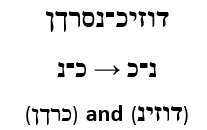The sum of 5 of the letters (דוזינ) is 77, and the sum of their square roots is a close approximation to 10 times the square root of 3 or 10√3, or 17.3285… Meanwhile, the sum of their squares is 2701, the numerical value of the 28 letters of the Torah’s first verse.  Once again, the splitting of the alef-bet giving way to the Creation of the Torah.

The sum of the squares of the other 5 component letters(כרךן) is 784,000 or 28² x 1000, yet another reference to the 28 letters of the first verse. Because 28² is (1000 – 216) we get a possible reference to the 216 letters of the 72 Triplets and the perfect 1000 sum of their square roots. We can juxtapose that with 17.3285 being suggestive of 173, the small gematria of the 42-Letter Name.

Why Cubes?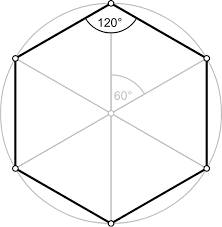We are professing that the origins of the Torah come from a higher intelligence and so we must assume that the Torah must also be viewed from a higher perspective or multi-dimensional one.  One way to do that from our admittedly limited point of view is to take the static numbers concretely given to us by the Torah and expand them geometrically to see what someone looking down on them might see, and what might otherwise be hidden from us. For example, a cube with 6 faces, 8 vertices and 12 edges or 26 outward facing elements when passing through our 2-d plane would appear to us as no more than a square at any moment in our linear-bound time. We would see only 1 face, 4 vertices and 4 edges, or 9 elements in total.  A line, or even string of numbers, passing through, would generate a single point or number.  The same goes for a string of 22 letters.

This is not as out there as you might think. We do this all the time unconsciously. When we look around us at any given moment, we see telephone poles, buildings, cars, people. In other words, any typical scene depending on where we are at the time. We cannot see behind or around the objects in our view stream; they may indeed be flat 2-dimensional cut-outs, yet our conscious minds fill in all the missing information and convert it into a 3-d world. The same happens with a painting or a photograph. Our minds will even do that with impressionist art or an abstract painting.  Our minds are filling in and creating new information based on what the artist programmed into his work, unseen algorithms, equations and relationships (spatial and otherwise). The same holds true for stories and novels. The writer gives us 100,000 words but our minds take it from there and fill in many times that in backstories, scenery etc. based on our individual and common consciousness and based on downloaded algorithms from the writer’s mind. A good writer can do that in fewer words. A poet can do that in very few.

We are a limited intelligence at the moment and yet capable of programming computers with intuitive AI capable of thinking for us and reacting millions of times faster than us. Some might say that is counter-intuitive and proves we are not that intelligent after all.  Regardless, ask yourself: What would a far more advanced intelligence do, transfer info to us in an enduring document that was limited to our capabilities of 3330 years ago, or knowing our trajectory, program it for us today or even for future generations?

One question to ponder: How were they (He) able to know, or to ensure, that that document endured perfectly intact, all 58 letters, words, and verses?

There are several ways to learn, one way used by bright inquisitive young minds and talented seasoned engineers alike is reverse engineering. When you are given a device or new technology and there are no books, lectures, YouTube videos or schematics to rely on, you carefully take it apart and draw your own schematics, then hopefully reassemble it correctly. Tiny protein machines inside the cells of our bodies do it all day long with our DNA. It would be only natural that an advanced intelligence would know that one day we would do that too with the Torah.  One could say they (He) made sure of it when we were given the exponential gematria codes to the alef-bet and the 42-Letter Name, an information transference device that we are only beginning to comprehend and utilize.One of the ways we can get an understanding or glimpse of what might be out there before it enters our reality and passes through it, is by observing its shadow. A cube casts the shadow of a hexagon.  The shadow cast by the Torah’s first verse combination 43 cube of 64 parts of 2701 cubic units each is a 37-unit hexagon. The total area of this resultant hexagon is 37 x 2701 = 99937, or (100,000 – 63). The shadow cast by the 42-Letter Name combinations 43 cube of 64 parts of 3701 cubic units each is also a 37-unit hexagon. This hexagon has a total area of 37 x 3701 = 136937 or (137,000 – 63). The difference between the two resultant hexagons is naturally 37,000.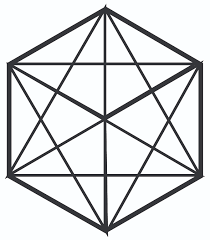As you can see with these diagrams, we can go easily from that hexagon to assorted other shapes including circles, triangles, diamonds trapezoids and 12-gonal stars inscribed in the hexagram and ones that circumscribe it.  There is a wealth of information that can be encoded by giving us a shape or a shadow.

These correlations should not be a great surprise to us as we have already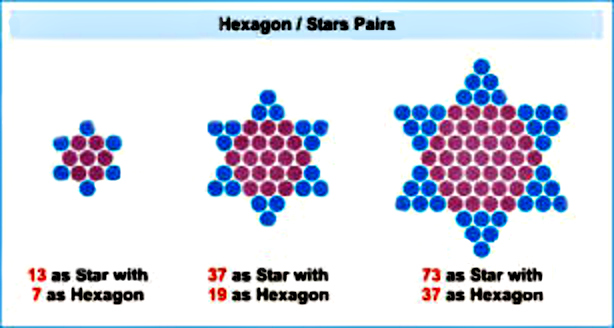seen that 37 units is the dimensions of the inner hexagon to the 73-unit Star of David since we started off with 2701, a triangular number that is the sum of the integers from 1 –73. No surprise, but still it was a planned geometric integration into the specific values of the first verse and how they would combine with each other.

Hexagon2701 = 37 x 2701 = 99937 = (100,000 – 63)

Hexagon3701 = 37 x 3701 = 136937 or (137,000 – 63)

The area of each of these two large derivative hexagons is off from a large round number by exactly 63, as in the 63 units of virtual space hidden in those 127 combinations, and as in the complement to 37 in that 100 – 37 = 63.  As we consider the role of 63 in these equations, we hark back to the relationship between the 670 paragraphs and Moshiach Ben David (424) whereby: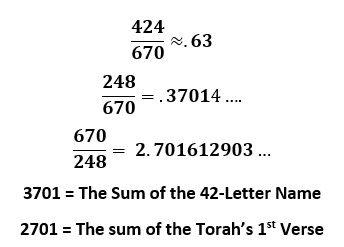Kabbalah has always had an explanation for this numeric relationship. Of the 10 Sefirot of the Tree-of-Life three (3) of them are of higher dimensionality than the other 7. These three (3) are Keter(620), Chochma (73) and Binah (67). The 7 lower sefirot are analogous to the 7 words of the first verse. The first two of the three (3) are extremely abstract to us, Binah (בינה) is the endless sea of energy, the place where the Oneness, the primordial Alef(א) exists and the place where the spiritual life-force that waters and energizes our world emanates. When the 4 aspects of the Tetragrammaton (יהוה) are spelled out, the first level is aligned with Chochma of numerical value 72 and the second one with Binah (יוד־הי־ואו־הי) of numerical value 63. So both 67 and 63 are directly associated with the concept of Binah. The third number or appellation associated with Binah is 1000, which partially derives from the 3 Yuds (י):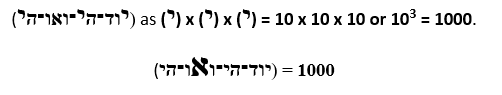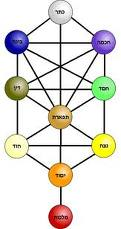So full disclosure about the kabbalistic understanding of some of these equations, the 7 lower sefirot complement Binah (63), hence 70 is the union of the 8 sefirot (7 + 63) and considered a completion. And the 8 or 8th level is Binah as in the 58 elements in the Torah.

We can ponder about 63, but 137, as in 137,000, is the gematria of “kabbalah,” meaning “to receive,” so we can wonder if the hexagon shadow based on the 7 lines of the 42-Letter Name is purposefully revealing an advanced technological way for us “to receive.” We do know that 137 is also directly connected to the Fine Structure Constant, but more on that later. For now, we can concretely see that 236864/137 = 1728.93 or rounding it off, 1729, the Taxi Cab number.

Taxi Cab Number

A couple months ago, as I was driving my daughter to school, we were behind a car with 1729 on its license plate, reminding me of the Hardy–Ramanujan Taxi Cab Number, which I then explained to her.  When Hardy went to visit Ramanujan in the hospital, he wanted to make small talk, so he mentioned that the taxi cab he rode in had the most boring number, 1729. Ramanujan replied that it was actually quite interesting, as it is the smallest number expressible as the sum of two positive cubes in two different ways (13 + 123 = 93 + 103). Yes, cubes again. But what Ramanujan did not know was there are exactly 1729 occurrences of the word value 26 in the Torah. That is the same 26 as in the 26 elements of a cube and as in the value (26) of the Tetragrammaton (יהוה), not to mention that the digits in (13 + 123 = 93 + 103) are (1 + 3 + 1 +2 + 3 + 9 + 3 + 1 +0 +3) = 26. Now that is indeed an interesting number!

Cubes on Steroids

The number 248 takes us all the way back to the beginning of this paper where we split the 22 letters of the Hebrew alphabet into two sets of 11 letters each, using the one and only “sequential” way to give us the 42 ,72, the Torah, and and phi proportions. While there was only one “sequential” way to do this, there were exactly 248 different 11 letter splits in total that summed to identical 625/870 proportions.  That was exactly 248 ways out of 705,432 different ways that the 22 letters could have been split into 11 letters.  And of those 248 ways, there was a subset that our one sequential split was part of, those whose 11 small gematria pairs summed to 541, the numerical value of Israel.  That subset had exactly 120 combinations. We know there were two significant men in the Torah who connected directly with G-d more than any other, one was Abraham and the other was Moses. We also know that the numerical value of Abraham is 248 and the lifespan of Moses was 120 years. We saw Moses’ birthdate, 2368, conspicuously woven into the combinations of the Torah’s first 7 words, and when we add his 120-year lifespan we get the year the Israelites entered the promised Land, Israel (541) immediately after Moses passed away, 2488 HC.

Yet another property of that original alef-bet split is found when we add in the sofit (final letter) gematria to the values already utilized.  Adding the final values of caf(500), mem(600) and pe (800) the alef(א) – shin(ש) side of our one sequential split goes from 625 to 2525, in keeping with the theme of multiples and the continuous squaring of 5.  Moreover, while the difference between the two sides would now be (2525 – 2470) = 55, the significance of which we will soon see.

To complete this symmetrical beauty in this splitting in two(2), we find that the sum of the integers from 1 – 100 divided by 2 = 2525, remarkable because 100 was the sum of the integers in the small gematria pairings that totaled 541 and  Israel (541) was the 100th Prime number. If it means anything at all, we just want to point out that 2525 – 2488 = 37.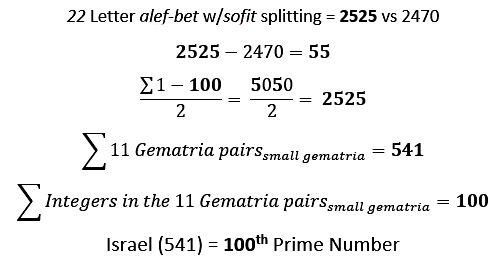It is interesting how this narration of the Torah and the fixed historical reference points follow this simple proportionate splitting of the Alef-bet.  It is almost as if the story, events, and timeline was created to fit the numbers.  It all had to have been known beforehand, thousands of years beforehand, or it would not have fit so precisely.

The sages told us that the Torah was written on 70 different levels and that the highest level was revealed through gematria. As we are seeing though, gematria is just a gateway to understanding.  Like a good video game, once we enter the gematria gate, there are many more hidden levels, each with hidden tools, clues, strategies to learn and find.  These numbers, 248 and 120, also come up in hypercubes and higher dimensional geometry and physics. The mathematics and physics here are beyond most of our readers so you can imagine what it must have seemed like to those of Moses and Abraham’s generations. Therefore, we are going to keep it as simple as possible and just point out the synergies between our Torah numbers and the multi-dimensional expansions of geometry.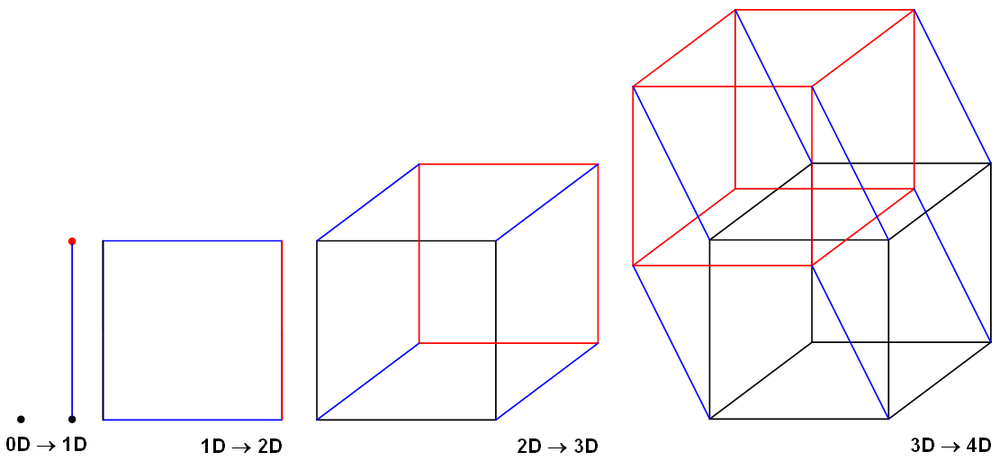After the lonely point, we expand into 1 dimension by drawing a straight line between it and a complementary point. The points are now known as vertices or the intersection of the lines, which will become edges as we expand into higher dimensions. We get into the 2nd dimension by drawing a square from our original line. Now we have 4 edges and 4 vertices and 1 face.  As we expand from there, each vertex or intersection will have a number of edges symmetrically coming off of it equal to its dimensionality. In other words, a 3-dimensional cube has 3 edges for each vertex, all symmetrically at 90-degree angles.  The number of vertices will always be 2n where n = the number of the dimension, as in the nth dimension, so our 3-dimensional cube has 23 or 8 vertices.

As we keep expanding from there into hyperspace our minds become inadequate for comprehending what these hypercubes look like and we need computers to process them for us. We can, though, project their vertices and edges downward, dimension by dimension, and represent them on a flat 2-dimensional surface.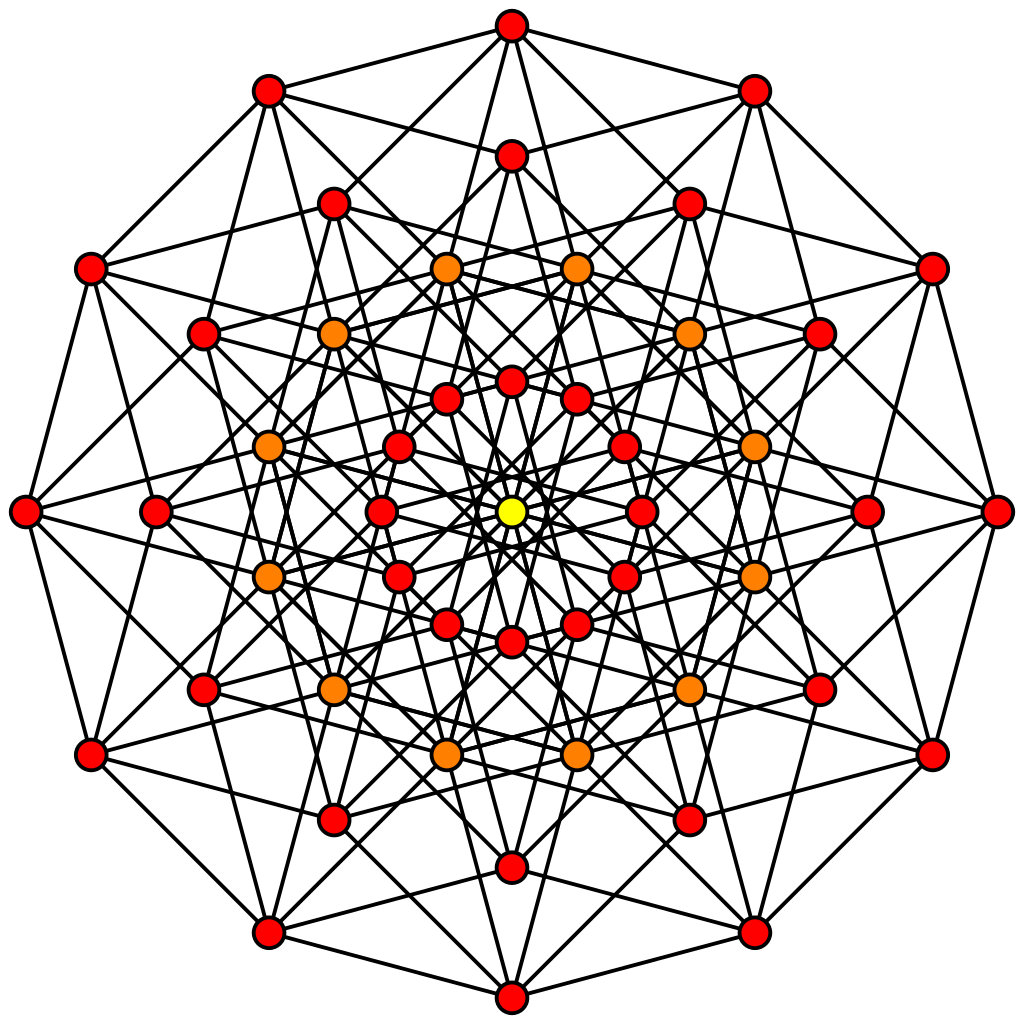So extending deeper and deeper into hyperspace we see that a 5-dimensional hypercube or polytope has 32 vertices matching the 32 pathways of the Tree of Life as noted by Abraham in his Sefer Yetzirah, and the 6th and 7-dimensional hypercubes have 64 and 128 vertices respectively, which matches up with the analysis we just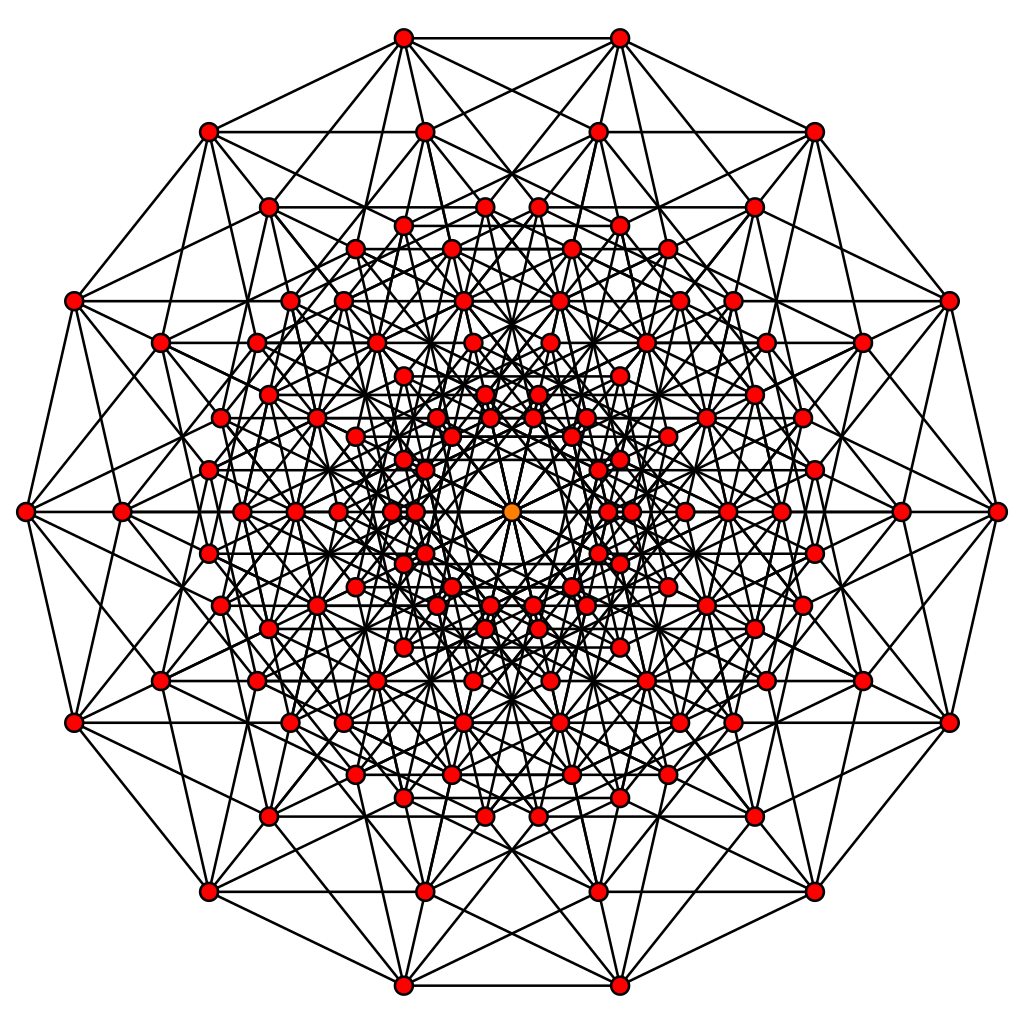did of the 128 combinations of the 7 first words in the Torah’s special first verse and then 64 times that each of the 7 words was used.  Since the 6-dimensional hypercube was the source for the 7-dimensional hypercube we are essentially dealing with one 7-dimensional hypercube whose 128 vertices each represent a different combination of the 7 words and 7 possible empty spaces, and which reflect the 64 times each of the 7 words was used.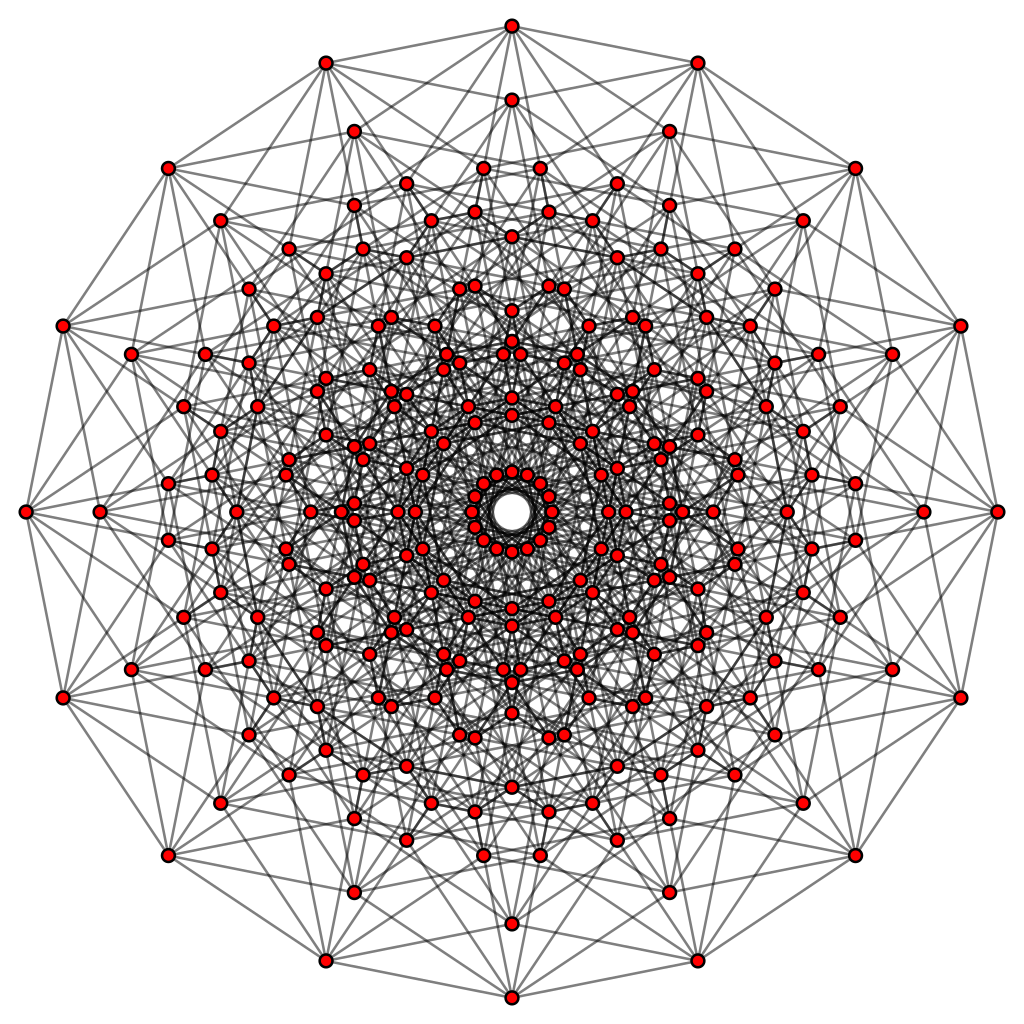Increasing in dimensionality we find that an 8-dimensional hypercube has 256 vertices, analogous to the numerical value of the Ark of the Covenant (256), where the Torah is stored, and a 10-dimensional cube has 1024 vertices, equating to the 1024, or 210, word values in the Torah.

An 8-dimensional hypercube is special, actually unique, in that while the number of vertices is 256 or 28, the number of edges is 1024 or 210, together that is 256 + 1024 = 1280 or 10 x 27. This equation works both for 8-dimensional hypercube and the 210 word values stored in the Ark (28). Moreover, 1024/256 = 210/28 = 4, possibly referential to the 4 letters of the Tetragrammaton(יהוה). This absolute ratio of 4 edges to each vertex is unique to the symmetry of the 8-cube, as the formula for edges for n-cube is n2n/2 which works out to edges = n/2 x vertices for an n-cube.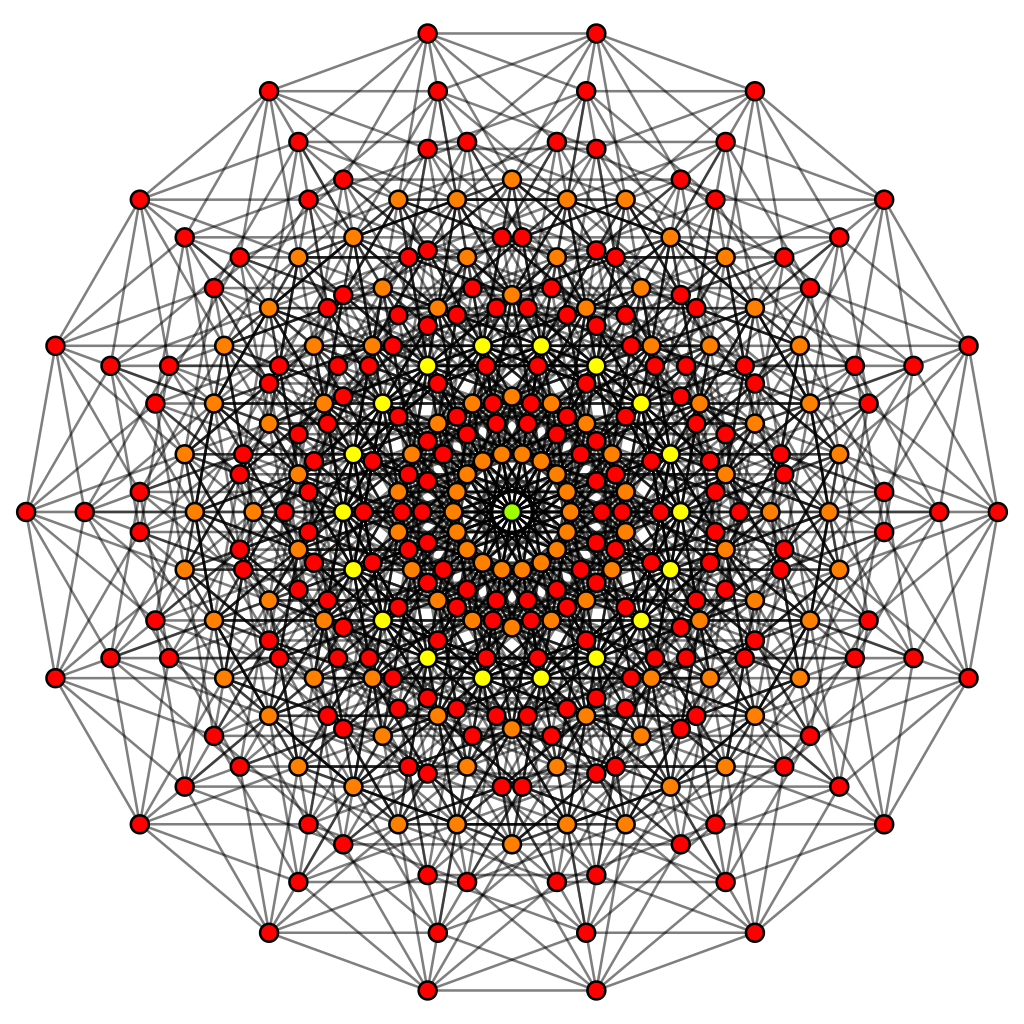Nevertheless, that was not why the 8-dimensional hypercube was so special. The number of faces of this particular hypercube equals 28 + 29 + 210 or 256 + 512 + 1024, which in turn equals 1792. What this means is that the number of faces in an 8-dimensional hypercube equals the number of vertices in the 8th, 9th and 10th dimensional hypercubes combined.  It also means the number of faces on an 8-dimensional hypercube equals the number of vertices in the full progression of the line, square, 3-cube, 4-cube, 5-cube, 6-cube, 7-cube and 9-cube and 10-cubes combined. That is actually wrapping the entire expansion in One. Then if these were not just points and planes in virtual space but had some assigned meaning to each one then there wouldbe virtually no limit what this technology could do or do for us. Is there meaning to 1792 being exactly 28 less than the 1820 Tetragrammaton(יהוה) in the Torah and those 28 Tetragrammaton(יהוה) add up to 728. Could it be that the 1820 Tetragrammaton(יהוה) directly connect with the 1792 faces of the 8-dimensional hypercube and or the number of vertices in the 28 + 29 + 210 hypercubes (8-cube, 9-cube and 10-cube) plus the 3-cube 4-cube and 2-cube(square).

Viewing the 58 elements of the Torah as an 8-dimensional hypercube, we can see how the Ark of numerical value 256, or 28,matches up with the 8-cube’s 256 vertices and how the 1024, or 210, word values matches up with the 1024 edges of the 8-cube.  This obviously brings up many questions.

E8 Lie group, The Torah and the Universe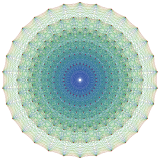The Kabbalists have always known the 8th dimension, which they called Binah, is special and is a level where all our physics collapses and where we get freed from entropy, thus releasing us from the constrains of time.  We will explain this later when we get into the physics of spherical time. Now, a giant step up in complexity is the E8 Lie group. The E8 Lie group is a 248-dimensional object, or 8 spatial dimensions with 248 symmetries.  Lie groups are a special set of symmetries and the E8 Lie group is one of the most complex shapes in our universe that shows up in both string theories and a newer possible fundamental theory of our universe.  This complicated circular webbed drawing is a very simplistic 2-D representation of a 3-d model of a 4-dimensional projection of an 8-dimensional object. It took a supercomputer years to model it.  It has 248 points of intersections.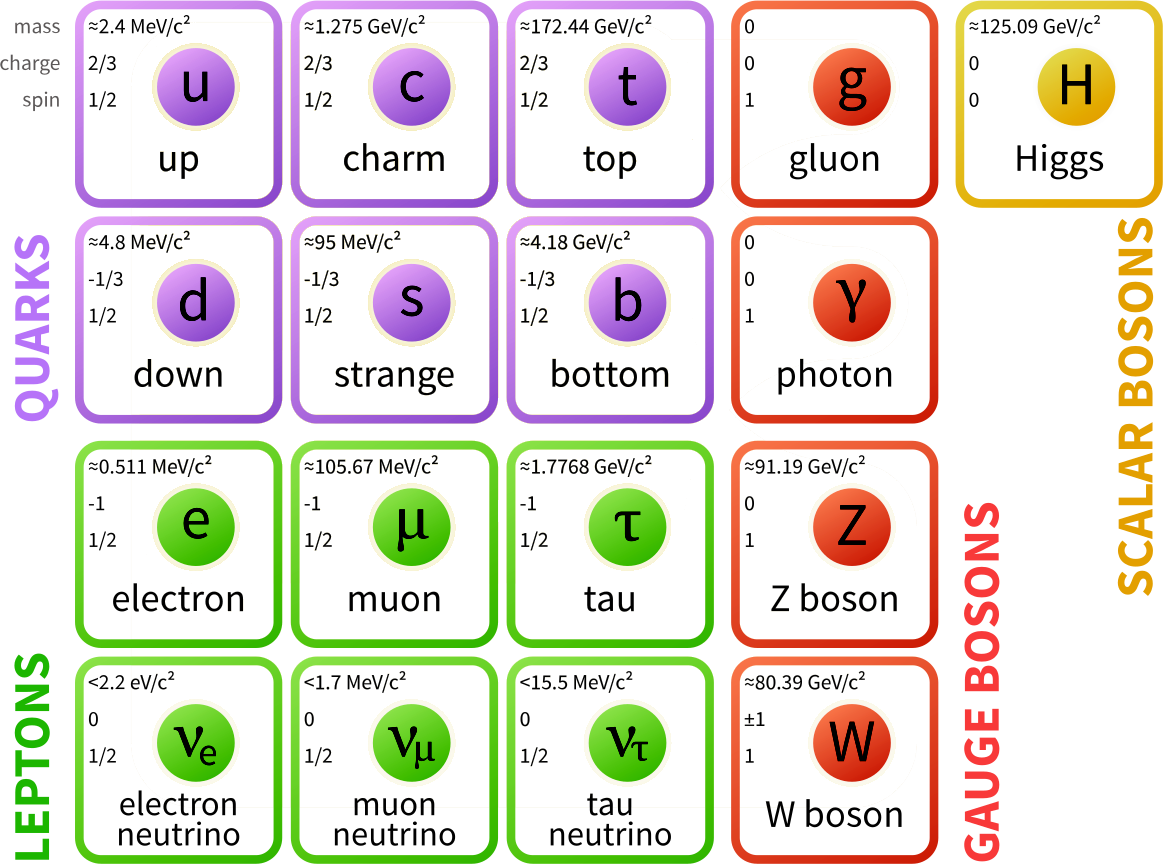There are 4 fundamental forces in our universe: The Strong force, the weak force, electromagnetism, and gravity. There are 12 fundamental particles, 6 quarks including the top quark with a mass of 173.07 GeV/c2, and 6 Leptons. Each of these has an anti-particle equivalent of equal mass for a total of 24 elementary particles plus the 4 bosons (Gluon, Proton, Z, and W) or force carrier particles. A total of 28. Each of the 28 particles has 8 assigned quantum numbers for a total of 224, leaving 24 dimensions or vertices of the 248 unaccounted for.   With the Higgs boson discovered in the last few years and a possible (probable) graviton, as yet to be discovered, that would leave 22 vertices unaccounted for. Hmm!

Is it possible that Abraham (248) was correct in that the 22 letters are essential and elementary building blocks for our universe? Is it possible that this trail of breadcrumbs we were left is guiding us in that direction?

Of Jacob’s 12 sons, Leah had 6 and the other 3 mothers had 6 more, analogous to the 12 fundamental particles (6 Fermions and 6 Leptons).  The 4 Bosons may be analogous to the patriarchs, Abraham, Isaac, Jacob and Joseph. Please note that even though Joseph was one of the 12 sons, he was replaced by his two sons as princes of the 12 Tribes, which freed him to be a Patriarch.  Add Moses and the final force, Moshiach, and the Torah aligns with the fundamental force and particles in our universe.  The kabbalists have always said the Patriarchs were more than significance personages in our history; they referred to them as energy forces so none of this is surprising.  At the moment, there is not much we can do with this right now, but with more understanding perhaps we can complete the equations using the numbers the Torah associates with them, such as ages and census numbers for the tribes.

In mathematics, E is the designation for several closely related exceptional simple Lie groups of dimension 248, and the same notation is used for the corresponding root lattice, which has rank 8.  The E8 lattice is far more complex than we have portrayed it. Each point in it is surrounded by 2160 8-orthoplexes and 17280 8-simplices. Each 8-orthoplex is a regular 8-polytope with 16 vertices, 112 edges, 448 triangle faces, 1120 tetrahedron cells, 1792 5-cells 4-faces, 1792 5-faces, 1024 6-faces, and 256 7-faces. You get the point; Very complex. Nevertheless, 2160 is not only referential to the 216 letters of the 72 Triplets, but also to the 2160-mile diameter of the moon, and 17280 is likewise referential to the 172864 total value of the 127 word combinations.

One last observation about hypercubes and the Torah’s first 7-word verse. We saw that the empty or null set of non-word values amongst the 128 total combinations totaled 64 x 7 or 448 units.  You may have thought we were pulling connections out of thin air, but a 7-dimensional hypercube has 128 vertices, 448 edges, 672 square faces, 560 cubic cells, 280 tesseract 4-faces, 84 penteract 5-faces, and 14 hexeract 6-faces.  The picture of a 7-dimension null hypercube within an 8-dimensional hypercube is taking shape before our eyes. Black fire on white fire, indeed.  This exists, and while invisible it is indelible, whether there is any connection between 448 and 2448 HC, the year the Torah was received on My Sinai, or not.

111

Steering away from the differential calculus of Lie groups and back to simple mathematics and the first verse of the Torah, the 1st and 3rd words add up to 913 + 86 = 999, or 9  times alef(אלפ); the 2nd, 3rd and 5th words add up to (395 + 401 + 203) = 999 as well; then the 3rd, 5th and 6th words sum to (86 + 395 +407) = 888; and finally the 3rd, 5th and 7th words add up to (86 + 395 + 296) = 777. Altogether these 4 different combinations that add up to multiple of alef(אלפ) equate to 33 x 111 or alef(אלפ).

Of the 127 combinations, 6 of them sum up to a multiple of 111 alef(אלפ), matching the 6 alefs(אלפ) of numerical value 111 that are found in this same first verse. The first multiple of 111 to be found amongst the 127 combinations is in the 10th highest combination value (2220) or (20 x 111), represented as 20(א).  The second one found is the sum of the first 5 words (913 + 203 + 86 + 401 + 395), which equal 1998 or (18 x 111), or 18(א).

Of those same 127 combinations, there are also 23 combinations that are multiples of 37.  These 23 combinations represent 18.10% of the total 127 combinations. So once again 181 comes up, the 42nd Prime Number, and the sum of the first 42 digits in the set of all Prime Numbers, and this time connecting back to the 181 digits in 111! (factorial).

The 5 That Don’t Jive

Just as this paragraph may seem out of place here, there is a seemingly incongruent time-line of 5 consecutive portions in the Book of Exodus: Yitro, Mishpatim, Terumah, Tetzaveh, and Ki Thisa, stretching from Exodus 20 – 34; from paragraph 54 to 130; and from the 10 Commandments to the second set of Tablets.  Just to put that into perspective, that span of the 5 portions that have 22 letters in their combined 5 Hebrew portion names is exactly 22,000 letters, 5788 words, 441 verses, 77 paragraphs and 15 chapters.  Right off the bat, the connection between the shift of 54 and 13 degrees and the 54th  – 130th paragraph at Mt Sinai of numerical value 130 is obvious.  Moreover, that 441 is the gematria of Emet, truth, and that there are exactly 1000 letters on average for each of the 22, is glaringly precise. Next, we note that these 5 portions represent 7.2% of the entire Torah, a possible reference to the 72 Triplets.  Then, while the total gematria of those 22,000 letters is 1733324, it is debatable whether the 173 reflects the 173 small gematria value of the 42-Letter Name in a similar way that the Top Quark having a mass of 173.07 GeV/c2 may or may not reflect it as well. Nonetheless, 1733324/26, the value of the Tetragrammaton (יהוה) is precisely 66666.308 and that was most probably planned for and designed that way.

In analyzing those 22,000 letters, we see that many of them reoccur very specific and significant numerical times. It would be natural for one of them to, even two, but of the 22 letters, at least 7 of them do. For instance, the letter Bet (ב) reoccurs 1024 or 210  times, the same as the exact number of different word values in the entire Torah, and the 1024 edges of the 8-dimensional cube. Both the letters Gimmel (ג) and the letter Tet (ט) each reoccur 137 times, as in the numerical value of kabbalah, meaning “to receive” and the Fine Structure Constant (1/137). Speaking of the Fine Structure Constant (1/137), when we sum up the cube roots of all 127 combinations, they total 1370.872. The letters Gimmel (ג) and the letter Tet (ט) are the only two letters of the Torah never found next to each other and they spell get (גט), “a bill of divorce,” a separation. The letter Chet (ח) reoccurs 616 times amongst these 22,000, and that is the numerical value of H’Torah, and is also the exact number of times Moses’ exact name (משה) is mentioned in the Torah. It is also the value of Yitro(יתרו), the first of the 5 portions. Then while the letterPe(פ) reoccurs 358 times amongst those 22,000 letters, as in the value of Mashiach(358), the letter Ayin (ע) reoccurs 954 times, as in digit #954 in Pi where we find the final of the three triplicate 5778 previously discussed.

Partitioning and more partitioning into meaningful subsets. Each value may have its own vertex and/or its own edge in either an 8-dimensional or 10-dimensional cube, and each one an intersection with so many more connections with other vertices and sub-regions, shapes, dimensions. It could take a supercomputer to model and reverse engineer it, begging the repeated question as to why were we given this magnificent instrument? And will we ever know how to utilize it properly? The answer may be up to us.

If, instead of having G-d’s Names all over the technology, it had alien markings, there is no doubt scientists would be pouring all over it, parsing it, studying its partitioning and symmetries, ordering its vertices, searching deeper and deeper for clues, answers, and a way to escape our reality. Do you not think the Creator of this unfathomably deep technological document and device did not know that?

Perhaps, in order to fully access this technology, we have to believe. Not in it, but in G-d, the Creator and in Oneness, just as it says in the Torah.

This abstract concept is not so far-fetched, welding an unseen force like belief and consciousness to hardwired physics.  Does a computer work without software? And even more abstractly, how can we explain the uncertainty principle and quantum entanglement? The truth is right now we cannot. They are observations we have made, and we have yet to find the knowledge to make sense of them.

There is super-determinism that tells us that whatever we actually observe was pre-determined all along, was pre-destined to happen. That does explain it, but it negates ALL free will and places each of us in a set movie in which we can only observe not alter.  That would negate any raison d’etre for our existence and make us an insignificant miniscule hiccup in a multi-verse universe that has vast other options, just not for us.

I am not saying that we have a special place in the vast universe, only that we were chosen to receive the Torah for a reason, not as a plot device in a movie.  We are not characters in a Pirandello play spontaneously awakening from our movie, searching for our author. Or are we? Either way, we found consciousness 5778 years ago and are just now learning what we can do with it.

The problem with quantum entanglement is that all our physics so far is predicated on Einstein’s theories that nothing can travel faster than the speed of light, not even if we were to find sub-photons or messenger photons. The reason this is an issue is that we have observed that if a change is made to a particle on one side of the universe an automatic corresponding change will occur in its paired particle on the other side of the universe and that cannot happen without significantly faster than light travel.  Unless, of course, there were forces at work we have yet to understand, or another dimension with different physics that connects everything using a different type of force entirely.

When something is unknown, invisible to our physical observations, and yet has some visible effect on our world (universe) we tend to call it spiritual.  At which point most people either ignore it or filter it through their personal and collective conscious belief systems.  The truth is we do not have a good grasp on consciousness either. No one actually knows what consciousness really is. Sure, we know our minds work through interlocking neural networks with feedback systems and loops, but beyond that, like the spiritual world, consciousness is an invisible force that is just there.

Given that, would it be so unfathomable that the forces of consciousness and the forces of the spiritual world might interact. And might there be tools that could facilitate and help us to consciously harness that interaction? Might the kabbalists be correct when they told us the 22 letters were conduits of that interaction, that the specific Names were just such tools enabling us to harness it, and that the Torah is both our instruction manual and motherboard to complete engagement?

G-d’s Covenant

Back to the technology itself. One additional point about the letter Pe(פ) of numerical value 80 in the Torah.  We have spoken about the obvious split in the 304,805 letters between the 4805 Pes(פ) and the other 21 letters. What we neglected to mention about the programming was that the collective value of those 4805 Pes(פ) was 384,400, whose square root is exactly 620, Keter (כתר). This is perhaps an indication why there are 80,000 – 52 words in the Torah. And perhaps this is reflected in the triplet 805 of the 304,805 letters, which is also the value of “The rainbow (הקשת)” in Hebrew, the sign of the Covenant G-d made with the Earth, the sign of the covenant between G-d and all flesh on Earth, according to the Torah in Bereshit verse 9:13. This occurred right after the flood (מבל) of numerical value 72, which begins with the letters (מב) or 42.  Strengthening these possible connections is the ordinal value of the rainbow (הקשת), 67, the same as the value of Binah (בינה), the dimension where those spiritual forces discussed above exist, the place, according to the kabbalists where the forces of our consciousness interact outside of physicality. G-d also refers to the rainbow as “my rainbow (קשתי)” whose ordinal value is 72.

A covenant with G-d is a Brit (ברית), associated with a splitting or severing, like G-d’s Covenant of Halves with Abraham and a Brit mila, the separation from negativity for an 8-day old male baby.  Certainly, this splitting or severing of Keter in the 304,805 letters is readily apparent.  What is interesting is that the square root (the splitting into two equal components) of 390,625 gave us 625, H’Keter, and the square root of the total value of those severed 4805 Pes(פ) gave us 620, Keter with a difference of 5 between them, as in 58.

G-d said to Noach(נח), of numerical value 58, about the rainbow that it was “a sign of the covenant ( הבריתאות).” The word “sign” is the same word for ‘letters” and the numerical value of this phrase is 1024 or 210, as in the precise 210 word values in the Torah.  A Covenant indeed!In that 4th line of the 42-Letters that we just drew the connection to the value 704, we find the word Brit spelled out in order (ברית).

Today is Lag B’Omer, the 5th day of the 5th, Hod of Hod. I hope everyone takes full advantage of the energy of R’Shimon Bar Yochai and of the power of this wonderful day.

Ezra

The final section of Part VI will follow shortly and we will get right into Bell Primes.

36 thoughts on “A Different Perspective”

1.Antonio says:

I only know that I know nothing.

Excellent job, Jeff, congratulations, Happy Lag Ba Omer for all.

We always have the jump of the Emunah

2.Stephen I. Ternyik says:

Excellent elaboration on Gematria. Congrats!

3.JJstar says:

BS”D

What can a person who is not great at quantum physics and mathematical equation take away from all of this wondrous knowledge? And how can even a simple minded person begin to comprehend just a tiny bit – i.e. like the kabbalistic equations for kindergartners? Thank you

4.Jeffrey Meiliken says:

Get what can, use the material to increase your belief, use the 42-Letter Name and understanding will come. The kabbalists were not physicists and most not mathematicians either, but they had belief and knew how to connect.

5.Moshe Friedman says:

At the beginning of the post it says that the number 127 is the 7th Mersenne Prime, a special class of primes.

The number 127 is also part of a special series of primes: 127 is the 31st prime, with in turn 31 also being a prime, the 11th prime, and 11 is also a prime, the 5th prime, and 5 the 3rd prime, 3 the 2nd prime, and 2 the 1st prime.

It is interesting that the 127th prime is 709, which is the next prime after the number 704 that you discuss in the post, and 709 = 704 + 5.

Also interesting is that 704 = 11 x 64, which resonates with more numbers in the post, and also 704 is a reflection of 407, another number discussed in the post, and together 704 + 407 = 1111.

It turns out that the number 1111 can be represented as Elef plus Alef, 1000 + 111, and also 1111 is a semi-prime, 1111 = 11 x 101, with 11 being the 5th prime and 101 being the 26th prime. Also in small gematria both 704 and 407 reduce to 7 + 4 = 11. (And it is interesting that in Yiddish the word עלף, pronounce “Elef,” means “eleven,” the number 11….)

That’s all for now, Good Shabbos!

6.Jeffrey Meiliken says:

Shabbat Shalom! Thank you for those comments. I with I would have caught that prime series myself which gives us Moshiach 358. Amazing catch! I am going to add it to the very next section-most grateful. BTW, 709 is the sum of the letters controlling the 7 planets according to the Sefer Yetzirah.

7.Peter says:

Heavenly Knowledge

Thankyou Ezra ! The amount of information, and the depth in to it — STUNNING !!

Will do a more “philosophical” and less mathematical comment here:

The name/word Chocmah (Wisdom) has a numerical value of 73. Binah (Understanding) has the numerical value of 67.

The 4th word of Genesis-Bereishith has the value 401. Combing that 401 with the 73 of WISDOM we have 474, the value of Da’ath (KNOWLEDGE).

The 6th word of Genesis is the same two letters as in the 4th word, Aleph and Tau, the beginning and end of the Aleph-Bet, with the “joining conjunctive Vau, which began as a pictogram of a Hook (two things hanging on a Hook) and a Nail or Spike (two things “nailed together). The value of Vau-Aleph-Tau is 407. Joining that value with Binah’s 67, we again produce the value of KNOWLEDGE (474).

WISDOM is Male, deep, almost subconscious. UNDERSTANDING is Female, associated with Building, and Words (Letters joined together). We do not KNOW something until we can put it into words. A Woman’s Mind, soul and spirit does what her body can do when it builds a baby. It takes the essence of the wisdom, the innermost DNA that is at its core, and then she “digests” what she wants and needs from it, duplicating it, and multiplying it, until it becomes a body of Knowledge, something that is a permanent “creation” of/from a human couple.

One of the “errors” of modern education and our mass media is that our “system” tends to bypass that male and female interaction, producing mental “clones”. We were meant to be unique couples, with each couple having a unique perspective on the KNOWLEDGE, as unique as the DNA of our children. That “bypassing” of the unique information between Male and Female began in Gan Eden, as the Nahash (Serpent) shortcuited the flow of information. The “agenda” is still there in our modern media and modern education.
The building of Knowledge, something that is done within and by a Woman is “a”, or “perhaps” the “High Point” in Creation. It is why a wife is “Queen” of her Home, and Queen of the Week, Queen of the Shabat, Queen of the 7th Day. (This will be more significant when we factor in the symbolism of the “8” as a portal into higher dimensions a few paragraphs ahead).

When we get it right, we get what is between those 4th and 6th words of the Torah – HEAVEN, Hashamaim, the only word of the 7 words in the Uni-verse, which does not contain one of those 6 Alephs. (I talk about Cricket and its 22 players relating to Torah later on. A lot of that “sports knowledge”, Cricket included, and our Shakespeare, came into the English speaking world from Venice directly (hence “OthellO’, Shylock and the rest of the “gang” from Venice, or from Venice via Prague. One of the main “conduits” was John Dee, the first “007”, Dee used to sign his letters to Queen Elizabeth I as “007”. He understood at least some of the significance of the 7 words in the first verse of Genesis. That was part of the reason he put the 7 in “007”.

Some of us males who have an orientation towards mathematics and equations have a great time with our numerical insights into the Torah. Other males who are less into the “numbers”, still do the same sort of thing through sport. Soccer with its 11 players per team, and two “Cheths” at each end of the field, is Torah converted into Sport. So is Croquet, where the Croquet Gates are a Cheth. The Gates are laid out in 3 columns. The Chinese symbol for 8 (Ba) is a Cheth, related to our European “h”, and the Greek letter “Eta”. They are symbolic representations of the 8 Dimensional Hypercube and E-8 Lie Group in this latest article from Ezra.

Americans who love their Baseball are probably unfamiliar with Cricket, the game played through the British Empire/Commonwealth. Cricket with its 22 players, its 22 yard Pitch, its 10 Wickets, and its 10 pieces of Wood, divided into two sets of 5 (Ten Commandments on 2 Tablets, 10 = 5 and 5, or Yod = Heh-Vau-Heh, ie the Tetragrammaton), is Torah and Genesis Chapter 1, laid out on a Sports Field.

All of that is Male, the Maths, the equations, the portals, the sports, the 10 wickets per innings etc. But what really counts is when that Male perspective gets digested, combined with a Female perspective, and Built into something Beautiful, into something new, into Knowledge. It is that beautiful Knowledge that is represented by Da’ath sitting above Tiphereth in the Tree. It is what builds our homes and our marriages. It is what is designed to keep us close and in love.

The Chinese (same written in all Chinese dialects/languages) symbol/pictogram for GOOD (“Hau” in Mandarin) is a picture of a Baby Girl in the House. Modern Chinese society with its “Male only one child per family insanity”, is a squillion miles from wht their ancestors knew, that the “high point” of what was in the home, was what grew in the heart of their girls.

The mathematics, or at least the volume of it in Ezra’s articles, and “our” comments, may seem formidable. But most of us can add 401 + 407 to get 808 or 8 times 101, which is the 26th Prime Number. That “8” is the Portal into a world above Time, above the 7 Days. That is why we use an 8 rolled onto its side, what Americans call a “Lazy 8” as our symbol for Infinity.

The “traditional” teaching is that the Letter Cheth began as a symbol for a Fence. That is not “fully” true. The Cheth is the GATE through the Fence, the Portal into what we have been cut off from.

When a baby is born, that new baby has an infinity of possibilities ahead of him or her. When new Knowledge is born, it also gives birth to an infinity of good possibilities. These articles are starting points for beautiful possibilities for those who are willing to “grow” them.

Ezra, THANKS for writing them . . . .

for

8.Peter says:

Seven Fold Manifestation of Spirit

Gong “back to the numbers”:

Those 3 words from Verse 1 of The Torah, words 4, 5 and 6, the pointers at Male and Female qualties wrapped on either side of Heaven, have the vlues 40, 395 and 407, which sum to 1203.

The Right Hand side of the Lower 42 Letter Name, i.e. columns 1, 2 and 3, hve the same 1203 value (471 + 417 + 315 = 1203).

The Rest of Verse One of the Torah has the value of 1498 (913 + 203 + 86 + 296.

Taking “Ruach” as having the vale 214 (200 + 6 + 8 = 214), we can see the rest of the verse as a 7 Fold expression of Spirit (Ruach). Kind of appropriate for something that is connected with a 7 Row Name.

9.Peter says:

should have read 401, 395 and 407 for the 1203 total in the previous post.

IBM 7040

Decades ago, I used to work on an IBM Mainframe computer, the Model Number being 7040.

I have (for many years now) suspected some of IBM’s former leadership of “dabbling in the dark arts”. So, I have wondered periodically if the IBM name (the 70th Triplet of the 72 Triplets if you translate Yod-Mem-Bet into I-B-M) and the 704 0 Model Number which looks so like the 704 “4th Row” of the 42 Letter Name were only coincidence.

Four Cubits
Four 17.6 inch Cubits (the “standard length” from which we get our 1760 yard mile) have the Length of 70.4 inches.

Four Cubits by the 4th Row ??

That might imply 7 Cubits over 7 Rows, or 123.2 inches – shades of a “Double” HaTorah (616 * 2 = 1232), either the Written and Oral Torah, or One Torah on Earth and another copy in Heaven (5th Word – the 5 “thingey” again !

Another way of looking at the Ruach 214 value (earlier post) is 206 with a gateway-portal (even earlier post), i.e. the 206 bones in a human body (ancien tradtion which combines those 206 bones with 42 other supporting structures to make up the 248 that has been so prominent in recent articles).

Looking at Ruach this way, the Human Body is meant to be translated through spirit into Heavenly territory – the “8 thingey” to go with the “5 thingey” in the 5 raised to the 8th power, 390625 Torah components etc.

10.Jeffrey Meiliken says:

Thanks Peter. By the way, this means that the left hand side of the 42-Letter matrix likewise necessarily = the 1st, 2nd, 3rd and 7th words of the Torah + 1000 or alef. Once again, astonishing! great work!

11.Peter says:

Thanks Ezra,

I often think of the cube of 12 (1728) in similar fashion — as an Aleph (rather than as an Eleph for 1000) attached to 728 to give us the 2nd line of the 42 Letter Name (729, i.e. the Cube of 9).

12.Peter says:

The “Fifth Column”

The 1792 in the article is Twice the value of the 5th column in the 42 Letter Name (896).

The 1792 is laso 28 (the numbers of letters in Verse 1 of the Torah) less than 1820, which is the number of times The 4-Letter Name occurs in the Torah.

13.Peter says:

Inside The Divine Matrix
There are 1024 “word values” in the Torah. Theose 1024 values are spread from 3 to 1500 (inclusive). That means that there are 474 empty “slot” spread out between 3 and 1500. (1500 minus the first two slots (values 1 and), means that the 1024 values sit within the range 3 to 1500, i.e. within 1498 possible values or “slots”. Subtracting 1024 from 1498 possible values, we have 474 “unused” word values.

That value, 474, is the value of the word Da’at or Da;ath, meaning KNOWLEDGE.

In some sense, this tells us that the Knowledge is in the spaces. This idea is consistent with the idea of the “White Fire”, the spaces around and between the Torah Letters (the Black Fire) are important

14.Peter says:

The Measure of The Divine Matrix
The 4-Letter Name (Tetragrammaton) occurs 1820 times in the Torah. The Divine Name (“Elokim”/Elohim) used in Genesis Chapter 1, occurs 42 times in the three books we call Exodus, Leviticus and Numbers.

“Wrapped around” those 42 occurences, we hve 156 times in Genesis, and 30 times in Deuteronomy that those names occur. These numbers (156 and 30) are the values of the names Joseph and Judah in Hebrew. They symbolise (among other things), the “two Messiahs), a Son of Joseph and a Son of David-Yehudah, wrapped around the 42.

The total of 156 + 42 + 30 is 228. So the total number of occurences of these two Divine Names across or through the Torah, is
1820 + 228 = 2048.

2048 is 2 raised to the 11th power, what we know in “Computer Speak” as 2 Kilobytes. The 2048 represents the corners or vertices of the 11 Dimensional Matrix in which our 10 Dimensional Universe “sits” or is contained.

If we tke the “slots” idea from my previou commnt, there were 474 empty slot within the 1024 Torah word values, and 550 (2 + 548) empty slots around the 1024 word values.
Tree of The Sephiroth
Between 1500 and 1820, there are 320 “slots” associated with the 4 Letter Name. Shades of “LeV (Heart) and the Tree of the Sephiroth expanded to 32 components within teach of the 10 “Top Level” Sephiroth.

322
There are also 2 “slots” associated with the 4-Letter Name “outside” of the other end of the Matrix, i.e. values or slots 1 and 2, before the value 3 for Ab or Av for father.
The “322” has significance from various perspectives including Number Series/Sequences, as well as being twice the value of Joseph’s name – and, of course, a rather well known university “fraternity or secret society that some of us may have heard of . . .

15.John Holleman says:
16.Dennis Pittenger says:

Hello, getting back to the issue of the tight linkage between 5778 and 2019 in the first thousand digits of Pi, and also the linkage between 2019 and the 42-letter name of G-d in the first thousand digits, I am in full agreement with you that this will prove to be the key that solves the puzzle (along with many other line of evidence from Biblical mathematics), however, I am starting to become concerned about the following: One of the evidences for 5778 is that Israel’s 70th anniversary fell in 5778 however, since 5 Iyar 5779/ May 10 2019 we are now into Israel’s 71st year. Do you have any thoughts on this?
Sincerely, Dennis

17.Jeffrey Meiliken says:

I cannot say except that the 72 Triplets is also referred to as the 70 triplets because 2 are the same. Something similar happened with the 70 members of the Sanhedrin.

18.Jeffrey Meiliken says:

Thanks for providing everyone with these links. They are very good though My French is no longer strong enough to follow Rav David Menaché. They will help with the significance and technology embedded in individual portions and sections of the Torah, which is very important. Right now, we are trying to expand our understanding and abilities beyond the bounds of this world and to do so we need to bounce back and forth from the micro to the macro. Like teh teaching of Kabbalah, it is not only an education, but a process. B’H we will succeed.

19.Jeffrey Meiliken says:

Thanks again Peter. 228 is Baruch and also Etz Chaim (the “Tree-of-life”). A secret of Baruch is that it is also 708, the upper 42-letter Name, a secret to be used in every blessing. I was going to warn you about 322, when I read the final line and saw that you were well aware.

20.Antonio says:

Excuse me, in reference to 322 comment that as I read the Master Friedrich Weinreb in his day, one of the greatest secrets of this number lies in the multiplication of 14 as a relation 1-4 with which the Torah is built by 23 as a symbol of the Jaiah 14 x 23 = 322

Shalom

21.Moshe Friedman says:

Ezra, thanks for your reply to my comment, and for saying you will include an idea from it in your next post.

At first I didn’t see the connection of the number 127 to Moshiach, gematria 358, but upon reflection I found that the sum of series of the 6 primes 127 + 31 + 11 + 5 + 3 + 2 = 179, and the number 179 is also a special prime, part of a series of primes, since 179 is the 41st prime, with 41 being the 13th prime, and 13 being the 6th prime.

The gematria of Moshiach, 358, is a semi-prime, with 1 factor being 2, the 1st prime, and 1 factor being 179, the 41st prime (as just said), and doubling 179 (with the concept of doubling being related to the splitting of the Alef Beis into matching sections, 11 + 11 = 22, or 2 x 11 = 22) gives 179 + 179 = 358, and 2 x 179 = 358, and therefore 127, resonating with 179, the additive sum of its related primes, when doubled or paired is 358, a hint to Moshiach (and the idea of doubling also a key aspect of Moshiach’s mission, as explained in many places).

There is also another hint to Name of 42 that is evident from the number 358 for Moshiach, since the ordinal numbers of the component primes factors of 358, i.e., the ordinal numbers of the primes 2 and 179, are respectively 1 and 41 (since 2 is the 1st prime and 179 is the 41st prime), and this yields the sum 1 + 41 = 42, hinting to the Name of 42 which is an inner spiritual force inside Moshiach that he will reveal to the world.

There are probably many other ways as well to see further indications of Hashem’s explicit Divine providence in these numbers and their relationships, in the roots of mathematics, in science, and of course in the Torah, and to understand that it is all one, since it all stems from the One Hashem.

———-

While on the subject, the sums of the intermediate numbers in the prime series culminating in 127 that sum to 179 are also significant in their own right:

Combining the first 2 primes in the series, 2 + 3 = 5, corresponding to the 5 Books of the Torah.

Combining the first 3 primes, 2 + 3 + 5 = 10, corresponding to the 10 Commandments, the 10 Sayings, and the 10 Sefiros.

Combining the first 4 primes, 2 + 3 + 5 + 11 = 21, which is the gematria of the Divine Name Ekeyeh, and also the first three letters Yud Heh Vov, 10 + 5 + 6 = 21, of the Name Hashem.

Combining the first 5 primes, 2 + 3 + 5 + 11 + 31 = 52, corresponding to 52 = 2 x 26, i.e., the doubling of the full Name Hashem, and also corresponding to the Name of 52, one of the four ways of fully spelling out the 4 letters Yud Heh Vov and Heh of Hashem.

Somewhat similarly for the series of primes culminating in 179:

Combining the first 2 numbers in this series, 6 + 13 = 19, and 19 (like 179, 41, and 13, but not like 6) is itself also a prime, the 8th prime, and 6 + 13 also hints to 6-13, i.e., the 613 Mitzvos, and in small gematria 6 + 1 + 3 = 10, and reduces to 1, representing the 10 Commandments and that Hashem is Echod/One.

Combining the first 3 numbers in this series, 6 + 13 + 41 = 60, which 60 = 4 x 15, with 15 being the gematria of the Name Kah, Yud Keh, corresponding to a double doubling of this Divine Name, and 60 also corresponds to the 60 letters of the Birchas Kohanim, and to the 6 Orders of Mishnah, and to the 60 Tractates of Talmud, and to the 600,000 souls of Israel. If only the primes in the series are counted, then combining 13 + 41 = 54, the special number Ezra discussed in the blog post.

———-

In the post it was pointed out that the number 127 is a Mersenne prime, and to provide a bit more relevant background, Wiki explains (and see there for more) that “Mersenne primes, of the form (2 ^n) – 1, are the primes 3, 7, 31, 127, 8191, 131071, 524287, 2147483647, 2305843009213693951, 618970019642690137449562111, 162259276829213363391578010288127, 170141183460469231731687303715884105727 …, and as of 2018, there are 51 known Mersenne primes, and the 13th, 14th, and 51st have respectively 157, 183, and 24,862,048 digits. As of 2018, this class of prime numbers also contains the largest known prime: M-subscript 82589933, the 51st known Mersenne prime.”

Regarding the Wiki entry for the number 127, the first thing noted about it is that it is a Mersenne prime:

“As a Mersenne prime, 127 is related to the perfect number 8128. 127 is also an exponent for another Mersenne prime 2127 – 1, which was discovered by Édouard Lucas in 1876, and held the record for the largest known prime for 75 years – it is the largest prime ever discovered by hand calculations, as well as the largest known double Mersenne prime. Furthermore, 127 is equal to 2^7 – 1, and 7 is equal to 2^3 – 1, and 3 is the smallest Mersenne prime, this makes 7 the smallest double Mersenne prime and 127 the smallest triple Mersenne prime.”

Among various other special properties of 127, Wiki also mentions that 127 is a centered hexagonal number, which Ezra has discussed in his posts, and of special interest it states that 127 is the first “nice Friedman number” in base 10, since 127 = -1 + 2^7, as well as binary since 1111111 = (1 + 1)^111 – 1 * 1 (see Wiki for details; as far as I know I’m unrelated to mathematician namesake).

Unmentioned in Wiki’s entry on the number 127 is that it is also in the category of a “super prime.”

Super primes are one of 75 subcategories of primes that Wiki describes on its “List of prime numbers” page. Incidentally, the first of these alphabetically arranged subcategories of primes are “Bell primes,” and coincidentally we are also currently discussing Bell numbers here).

According to Wiki, “Super-prime numbers (also known as higher-order primes or prime-indexed primes) are the subsequence of prime numbers that occupy prime-numbered positions within the sequence of all prime numbers. The subsequence begins 3, 5, 11, 17, 31, 41, 59, 67, 83, 109, 127, 157, 179, 191, 211, 241, 277, 283, 331, 353, 367, 401, 431, 461, 509, 547, 563, 587, 599, 617, 709, 739, 773, 797, 859, 877, 919, 967, 991…”

———-

It comes out, that what I wrote about in my previous comment – “127 is part of a special series of primes: 127 is the 31st prime, with in turn 31 also being a prime, the 11th prime, and 11 is also a prime, the 5th prime, and 5 the 3rd prime, 3 the 2nd prime, and 2 the 1st prime” (and similarly in a my past comments with other examples of prime numbers is related series) – that these special primes are considered to be “super primes” (with the exception of 2, since its prime-numbered position is 1, and technically 1 is not included as a prime).

Even more, the super primes we have discussed are part of a series of super primes, and in this case, the series of super primes related to the super prime 127 is unique in that the series starts with 2, the 1st prime, making it what might be called an initial, primary, or origin-based series super prime. (I have not had time to investigate if these categories of super primes have been discussed as of yet in Wiki or elsewhere.)

The first 15 numbers in the origin based series of super primes that I have calculated with the help of online programs are: 2, 3, 5, 11, 31, 127, 709, 5381, 52711, 648391, 9737333, 174440041, 3657500101, 88362852307, 2428095424619, and so on.

In contrast, the super prime 179 – aside from being the sum of the 6 super primes in the series 2 through 127 – is also, together with (6) and 13, and 41, a part of a series of super primes, only the series it belongs to does not start from the 1st prime, or from any prime number, but rather from 6, which is only a semi-prime, the product of 2 x 3.

It is significant that in its entry on 127, Wiki includes the idea that the Torah mentions the number 127 prominently in connection with the 127 years of the life of Sarah, and the 127 countries ruled over by Achashveirosh and by Queen Esther, and these have been discussed before in this blog and in the comments as well.

———-

Another fascinating aspect of the number 127 is that 101 is the 26th prime, and 101 + 26 = 127, and this illustrates yet another connection to the Name Hashem, gematria 26.

This is an example of another special series of numbers that can be generated from primes, where the rule is to sum the primes themselves with the ordinal number of their position in the set of primes (I do not know if this series of prime-based numbers has been previously discussed or classified).

Calculating this rule for the first 11 primes generates the following numbers: (2 + 1 =) 3; (3 + 2 =) 5; (5 + 3 =) 8; (7 + 4 =) 11; (11 + 5 =) 16; (13 + 6 =) 19; (17 + 7 =) 24; (19 + 8 =) 27; (23 + 9 =) 32; (29 + 10 =) 39; and (31+ 11 =) 42.

It is fascinating to see that the first 3 numbers in this series are 3, 5, 8, and like the numbers at the beginning of the Phi number series that was discussed in past posts, this is another hint to the number 358, the gematria of Moshiach.

The following is a list of such numbers I generated through the 101st prime. In each equation the first number is the regular prime number, the second number added to it is its index or ordinal number in the set of primes, and the third number is the sum of the first two, the composite number of the prime number and its ordinal number in the set of primes. It is relatively easy to spot many significant numbers that are thus generated.

For clarity the prime ordinal numbers are highlighted, and likewise for comparison the primes among the composite numbers (using the * sign as highlighting for this version). The special primes generated this way are: 3, 5, 11, 19, 79, 101, 113, 127, 163, 173, 223, 271, 383, 419, 431, 503, 571, 599, 641…:

2 + 1 = 3*
3 + 2* = 5*
5 + 3* = 8
7 + 4 = 11*
11 + 5* = 16
13 + 6 = 19*
17 + 7* = 24
19 + 8 = 27
23 + 9 = 32
29 + 10 = 39
31+ 11* = 42
37 + 12 = 49
41 + 13* = 54
43 + 14 = 57
47 + 15 = 62
53 + 16 = 69
59 + 17* = 76
61 + 18 = 79*
67 + 19* = 86
71 + 20 = 91
73 + 21 = 94
79 + 22 = 101*
83 + 23* = 106
89 + 24 = 113*
97 + 25 = 122
101 + 26 = 127*
103 + 27 = 130
107 + 28 = 135
109 + 29* = 138
113 + 30 = 143
127 + 31* = 158
131 + 32 = 163*
137 + 33 = 170
139 + 34 = 173*
149 + 35 = 184
151 + 36 = 187
157 + 37* = 194
163 + 38 = 201
167 + 39 = 206
173 + 40 = 213
179 + 41* = 220
181 + 42 = 223*
191 + 43* = 234
193 + 44 = 237
197 + 45 = 242
199 + 46 = 245
211 + 47* = 258
223 + 48 = 271*
227 + 49 = 276
229 + 50 = 279
233 + 51 = 284
239 + 52 = 291
241 + 53* = 294
251 + 54 = 305
257 + 55 = 312
263 + 56 = 319
269 + 57 = 326
271 + 58 = 329
277 + 59* = 336
281 + 60 = 341
283 + 61* = 344
293 + 62 = 355
307 + 63 = 370
311 + 64 = 375
313 + 65 = 378
317 + 66 = 383*
331 + 67* = 398
337 + 68 = 405
347 + 69 = 413
349 + 70 = 419*
353 + 71* = 424
359 + 72 = 431*
367 + 73* = 440
373 + 74 = 447
379 + 75 = 454
383 + 76 = 459
389 + 77 = 466
397 + 78 = 475
401 + 79* = 480
409 + 80 = 489
419 + 81 = 500
421 + 82 = 503*
431 + 83* = 514
433 + 84 = 517
439 + 85 = 524
443 + 86 = 529
449 + 87 = 536
457 + 88 = 545
461 + 89* = 550
463 + 90 = 553
467 + 91 = 558
479 + 92 = 571*
487 + 93 = 580
491 + 94 = 585
499 + 95 = 594
503 + 96 = 599*
509 + 97* = 606
521 + 98 = 620
523 + 99 = 624
541 + 100 = 641*
547 + 101* = 648

In total this list has 101 primes, with 26 primes in the prime ordinal index, and 19 primes in the composite sums.

The opening note about this was that 101 + 26 = 127. A closing note for now is that together with 19 for the number of thus generated primes, 127 + 19 = 146, a semi-prime with factors 2 x 73, with 2 being the 1st prime and 73 being the 21st prime, and adding 1 + 21 = 22, perhaps hinting to the 22 letters, which in Hebrew the letters also function as numbers.

[For further reference the next 26 primes (prime ordinal numbers 102-127) are: 547, 557, 563, 569, 571, 577, 587, 593, 599, 601, 607, 613, 617, 619, 631, 641, 643, 647, 653, 659, 661, 673, 677, 683, 691, 701, and 709.]

22.Antonio says:

Ohr Pnimi …. 26.
Ohr Makif……21.
Ohr Yashar…..1820. Appearances YHVH en la Torá.
Ohr Jozer……107. Appearances HVHY en la Torá. (Invested)
1927 …….41×47 (21+26)……41 = 14 Prime. 1/4
Shalom

23.Antonio says:

Anoki Ejad……Alef….107.007 letter in Torá.
Elokim Eheieh…..86+21= 107
Anoki HaShem….81+26 = 107
Shalom

24.Peter says:

Seeds Of Miraculous Change
SO MUCH great stuff coming through in both the articles and the comments.
A Tree or plant IS a miracle. A little seed absorbs things from the dirt around it and grows into something beautiful that produces food, oxygen, flowers, colour . . . .

Etz (Tree) is spelled with the 16th and 18th Letters of the Alphabet, i.e. Ayin-Tsadhe. That is a representation of Phi, 1.618, the Golden Ratio that has featured so much in these articles.

Lots of great seeds turning into plants and trees here . . . . we are traveling through time, “micro to macro”, through physical, spiritual and mental space, from the 3-D world to the 8-D and 10-D as we climb (or try to climb !) through from a zero point inside us to exotic hypercubes . . . .

What we are doing, absorbing this material as we read it and process it, is the start of something big and something new. When we step back a little from all the mathematics and the science, we can see that we are in the middle of something that is uniting several languages and cultures, and several of the world’s spiritual traditions n-nalmost all of which had their source and roots (Trees again!) in Torah (or Torah concepts).
Gracias/Danke Antonio and “Todah” Moshe for the input/concepts – will keep me (and probably many others) busy for a while “absorbing” it all.

Collective intelligence working on something produces something bigger than just a sum of the parts. Going back a few articles, Era’s typing “error” regularly flipped the letter order to put Yod and Aleph together in the first word of the Torah. My hunch was/is that it was a subconscious “flip”, reflecting the idea of applying measures of Quality and Quantity, Words and Numbers (represented by Aleph and 10(Yod)) to the Matrix (ReSheT)bsplled Resh-Shin-Tau. We are In the image of God”, our minds do what the Divine Mind does, and we are (5778/2019 and all that) starting to get a whole lot better at it.

25.Antonio says:

666 = 6(3) + 6 (3) + 6 (3) = 648
648 = 6(3) + 4 (3) + 8 (3) = 792
792 = 7 (3) + 9 (3) + 2 (3) = 1080
1080 = 1 (3) + 0 (3) + 8 (3) + 0 (3) = 512
512 = 5 (3) + 1 (3) + 2 (3) = 15

153 Fishes . Yehohanan 21.11 = Sigma 17 = TOB = טוב

(3) Elevated to the cube.
As the light comes from the dark…

Shalom

26.Antonio says:

Sorry
512 = 5 (3) + 1 (3) + 2 (3) = 153

Shalom

27.Antonio says:

3.1415926535 = first 10 decimals of Pi

3.14 (2) = 26
Counting from first 1 from 3 to 3 = 1565
3. 1415926535 (2) = 232 = Expansion YHVH in 4 worlds = AB, SaG, MaH, BoN
232/4 = 58 = JeN = NoaJ
5 (8) = 390.625
8 (5) = 32768 = Add 26
Shalom

28.Peter says:

248 Fundamental Particles
Scientists at CERN and in other research centres are trying to identify all 248 particles that match with the E-8 Lie Group “Model” / Theoretical Model.

The idea that 22 of those particles are really the 22 Letters of the Aleph-Beit is one of the most stunning things in this article. That means that our science is ALREADY “in higher dimensions”, even if the scientists would get fired if they ever published that information in a “peer reviewed journal”??

The “real science” is happening on a level way above what gets circulated around university physics departments??

“Yahoo that we have these articles!” Todah Rabah to their brilliant author!

29.Moshe Friedman says:

My above (May 27) comment ended parenthetically, “For further reference the next 26 primes (prime ordinal numbers 102-127) are: 547, 557… 709.”

Correction: Prime number 547, with prime ordinal number 101, was already included in the last line of the summation table, 547 + 101* = 648. The extra reference should have begun with the 102nd prime, 557.

Since by Hashgacha Protis this focuses attention on the prime 557, here it is fitting to add that 557 + 102 = 659, and 659 is also a prime, the 120th prime. The prime ordinal number 120 has multifaceted significance and seems to resonate with the associated prime ordinal number 102.

Interestingly 659 + 120 = 779, and since 659 and its associated prime 557 have multiple 5’s, it seems we can join the idea of at least of these 5’s to 779 and get 5779, our current special Hebrew calendar year, a year of Moshiach, and as I pointed out in a previous comment, 5779 is also prime, the 758th prime.

[Just for fun, the sum of the primes 547 + 557 = 1104, the sum of their ordinal numbers 101 + 102 = 203 (the gematria of the first 3 letters of the Torah, Beis Reish Alef, and the 6th Bell Number, now being discussed in the blog), and the sum of 648 + 659 = 1307 (the 234th prime).]

30.Moshe Friedman says:

While this post starts with discussion concerning the first verse of the Torah, with its 7 words and 28 letters, it is also important to realize and bear in mind, especially now as we prepare for the upcoming holiday of Shevuos celebrating the giving of the Torah, that in the Kabbalah Secrets discourse on “The 10 Commandments” and elsewhere it is explained that:

The verse that immediately precedes and introduces the 10 Utterances, Exodus 20:1, וידבר א-ל-ה-י-ם את כל הדברים האלה לאמר, “God spoke all these words, saying:” has 7 words and 28 letters, just like the Torah’s first verse.

Is seems very far fetched to think that this is just a “coincidence,” and most certainly there is exceptional Divine Providence and Planning involved, especially since the Divine Name Elokim occurs in each of these special verses, and as our sages teach in many places there there is a direct link between the “10 Sayings” that created the world and the “10 Commandments.” There are many aspects of this connection that have already been revealed, and they should be reviewed as much as possible, and probably there are much more interrelated ideas that are still to be revealed.

[Also as a reminder, as explained in a previous comment, this year will be the 3331rd anniversary of Matan Torah, and 3331 is a prime number, the 470th prime.]

31.Jeffrey Meiliken says:

Many thanks for this, Moshe. I’m sure there is a lot in this verse w/2 of the words and thus 7 of the letters the same as Bereshit 1:1

32.Moshe Friedman says:

Ezra, it is your revelations in “The 10 Commandments” (linked on the sidebar) and in general that deserve the most thanks! There are so many amazing and stunning ideas you have shared, some of them astonishingly complex, that I’m still only beginning to scratch the surface of.

The correspondence between the Torah’s first verse, Breishis Bora Elokim Eis Ha’shomayim V’eis Ho’oretz, and the first verse of the 10 Commandments (Utterances), Vayedaber Elokim Eis Kol Hadevorim Ho’eileh Leimor, each with the exact same count of 7 words and 28 letters, is a relatively simple concept to grasp, and it is probably deserving of another post in and of itself, together with many other basic correspondences that are evident interconnecting the Torah’s account of Hashem’s Creation of the world and the Torah’s account of Hashem’s giving of the Commandments that could be explained.

———-

Here let me just add for now that the number 7 is a prime number, the 4th prime, and 7 x 4 = 28, and thus the average word size for each of these primary verses is exactly 4 letters long, perhaps hinting to the Tetragammaton, the special 4 letter Name of Hashem.

The sum of 7 + 28 = 35, which is the same as 7 x 5, and 5 too is a prime number, in fact it is a super prime, since it is the 3rd prime, and 3 is likewise prime, the 2nd prime, and 2 is likewise prime, the 1st prime, and thus 5 is not just a super prime, it is part of a series of super primes.

When 7 and 28 are doubled, by combining these 2 primary verses, then the respective sums of words and letters are 14 and 56, which sum to 14 + 56 = 70, and 70 is the same as 7 x 10, with 10 being 2 x 5.

It is possible to say that these numbers hint to the Two Tablets of the Covenant with the 10 Utterances divided into 5 Utterances on each Tablet. The First Tablet lists Utterances that stress the relationship between man and G-d, which in general expresses the theme of the Giving of the Torah on Mt. Sinai, i.e., between man and G-d, while the Second Tablet lists Utterances that stress the relationship between man and his fellow man, which in general expresses the theme of the Creation, i.e., and how man should interact with humanity and with his fellow creations .

———-

Last year the number 70 was emphasized, since it was the 70th year since the rebirth of the modern state of Israel. The number 70 as just explained hints to the completion of these two principle verses of the Torah, and surely the idea of this completion continues this year.

In addition, this year is the 71st year, and it is interesting to note that like the numbers 5779 (the 758th prime) and 3331 (the 470th prime) mentioned above, the number 71 is also a prime, the 20th prime.

Yesterday was Yom Yerushalayim, Jerusalem Day, and just to add something about that, the commemoration of the unification of the Holy City under Jewish control in 1967/5727, and when the Temple Mount returned to our hands:

It is interesting that the number 1967 has one digit that is a 7, and the digits 1 and 6 can be combined to make another 7. Also 1967, although not prime, is a semi-prime, the product of 7 x 281 = 1967, and that resonates with the idea of 7 words and 28 letters, since 281 is 28 x 10, plus 1 for the Kolel [It is also interesting that 281 is the 60th prime.].

Incidentally 1967 may be viewed as 19-67, and 19 is prime, the 8th prime, and 67 is prime, the 19th prime, and thus 67 is a super prime, since its prime ordinal number 19 is itself prime, the 8th prime, and the sum of these prime ordinal numbers 19 + 8 = 27, and with 1 for the Kolel equals 28.

The number 5727 has two digits that are 7, and the other two digits 5 and 2 also sum to 7, for a total of 3 x 7. [The number 5727 is non-prime, having prime factors of 3 x 23 x 83 = 5727, and these 3 primes sum to 3 + 23 + 83 = 109, which 109 is a prime, the 29th prime.]

It is possible to take the 2 x 7 in 1967 and the 3 x 7 in 5727, and sum them to 5 x 7 = 35, which is the same as the sum of 7 + 28 = 35, as explained above, and therefore these years when Jerusalem was unified also hint to both the first verse about Creation and the first verse about the Giving of the Torah.

This year we celebrate the 52nd anniversary of the return of Jerusalem to Jewish sovereignty. It is possible to say that the small gematria of 52 is 5 + 2 = 7, resonating with the idea of 70 years, and the 7 words in the two primary verses discussed. Also 52 = 4 x 13, hinting to the completeness of the unification (Echod, One, gematria 13) on 4 levels, corresponding to the 4 letters of Hashem’s Name, and also 52 = 2 x 26, i.e., a double hint to the Name Hashem gematria 26, and perhaps hinting to a fulfillment of Hashem’s plan both in the Creation and in the Giving of the Torah.

Kein Teyeh Lonu, Bimharo B’yomeinu!

33.Jeffrey Meiliken says:

Thank you. I’ll finish reading and give your comments the attention they deserve first chance I get. Meanwhile, I noticed, 5779 + 3331 = 9110

34.Moshe Friedman says:

Every connection we make leads to several more…

Regarding 9110, it is 10 times 911, the gematria of the word ראשית, Reishis, “Beginning” (as in B’reishis, “In the Beginning” at the start of the Torah), and it is the 156th prime, and together with 71, the sum is 5779 + 3331 + 71 = 9181, which is also a prime, the 1138th prime. Again all these numbers lead to further connections…

———-

Regarding Yom Yerusholayim, it may be added that it is celebrated on the 28th of the Hebrew month of Iyar, and the date 28 corresponds to the 28 letters in each of the two first verses Breishis Bora and Vayedaber Elokim.

Also the 28th of Iyar is the 43rd day of the Omer, and the number 43 is prime, the 14th prime, with the prime ordinal number 14 corresponding to the 2 x 7 = 14 words in these two first verses when added together, and with 14 also being the gematria of דוד, Dovid, King of Israel, who conquered Jerusalem and set it as our eternal capital.

Also the sum of 28 + 43 = 71, bringing out a special resonance of Yom Yerusholayim unique to this year, the 71st year since the reestablishment of the state of Israel.

———-

Just one more brief related teaser. It is possible to connect the first verse of Shma with the first verse of Breishis and the first verse of Matan Torah, i.e., with 7 words and 28 letters:

The verse שמע ישראל יקוק אלקינו יקוק אחד, Shma Yisroel Hashem Elokeinu Hashem Echod, Hear O Israel Hashem our G-d Hashem is One, consists of 6 words and 25 letters, and to this we may combine 1 more word of 3 letters to sum to 7 words and 28 letters. This may be done in any one of several ways:

By adding the 3 letter word אמן, Amen, that may be said just before the Shma in answer to the blessing before the Shma said out loud by the Chazan, and also the initials of א-ל מלך נאמן, Kel Melech Ne’emon, “G-d, King, Who is trustworthy,” customarily recited by some just before the Shma.

By adding the 3 letter word אמת, Emes, “True,” which is a word said immediately after the 3 paragraphs of Shma.

Or by adding the 3 letter word and Divine Name ש-ד-י, Sha’da’i, which as I explained at length in previous comments is intimately connected with the Shma.

Note too that our sages connected the 10 Commandments to various hints in the Shma, and Bli Neder this will be explained further at another time.